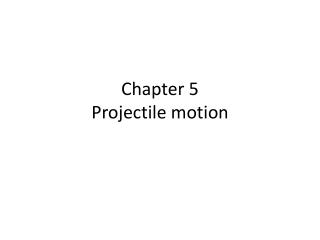DownloadDownload PresentationChapter 5 Projectile motion

# Chapter 5 Projectile motion

Download Presentation## Chapter 5 Projectile motion

- - - - - - - - - - - - - - - - - - - - - - - - - - - E N D - - - - - - - - - - - - - - - - - - - - - - - - - - -
##### Presentation Transcript

1. Chapter 5 Projectile motion

2. 2.Chapter 4: [linear motion]straight line motionthat was ONLY vertical or ONLY horizontal motion

3. 3. Chapter 5: looks at motion that followsa diagonal path or a curved path

4. 4. When you throw a baseball, it travels in an curved path.

5. 6. vertical motion is not affected by the horizontal motion. And the horizontal motion is not affected by the vertical motion.

6. 7. Observe: a large ball bearing is dropped at the same time as a second ball bearing is fired horizontally.

7. What happens?

8. Remember adding 2 perpendicular vectorshorizontal and vertical vectors.

9. 8. Remember: When we add perpendicular vectors we use Pythagorean theorem to find the resultant.

10. Boat in a river

11. Traveling up and down stream

12. 10. How fast will a boat drift downstream?

13. 11. Vtotal = Vboat + VcurrentVtotal = 0 + 8 = 8 m/sec

14. Now using the motor…

15. 12. Suppose the motor moves the boat at 15 m/sec.

16. 12. Suppose the motor moves the boat at 15 m/sec.how fast will the boat travel downstream [with the current]?

17. 13. Using motor with current: Vtotal= Vboat + Vcurrent

18. Total velocity traveling downstream: Vtotal= Vboat + Vcurrent= 15 + 8 = 23 m/sec 

19. 14.Using motor against the current: total velocity traveling upstream [AGAINST the current] Vtotal = Vboat –Vcurrent

20. 15. Going upstream: Velocity of current and boat are opposite directionsVtotal = Vboat –Vcurrent

21. Vtotal = 15 -8 = 7 m/sec 

22. Crossing the river

23. 16. If there was no current, how many seconds for the boat to travel 120 meters to reach the opposite side?

24. time = distance velocitytime = 120 m 15 m/sectime = 8 seconds

25. But there if is a current, what happens when you try to go straight across the river from A to B?

26. 18. If there is a current, The boat still crosses in 8 seconds, but it lands downstream at point C

27. 19. Add the perpendicular velocity vectors add to find the resultant velocity

28. The triangles are similar:

29. 20. In this example, Every second the boat travels 15 meter in the x direction IT ALSO TRAVELS 8 meter in the y direction

30. Velocity = distance timeso distance = velocity X timedistance = 8 m/sec X 8 sec = 64 m

31. 21) If you want to go from A to B instead, you must point the boat diagonally upstream to compensate for the current.

32. 22) Planes are affected by the wind the same way

33. 23) Head wind: slows the plane[opposite direction]

34. 24. Tail wind: speeds the plane up [same direction]

35. 25. crosswind: blows plane off course [wind perpendicular to direction of plane]

36. Break Vboat into VxandV y components

37. Use pythagorean theorem to find Vx.

38. How many seconds to cross?Velocity = Distance/timeT = D/V

39. How many seconds to cross? From A to BT = D/VT = 120/12.7 T = 9.4 sec